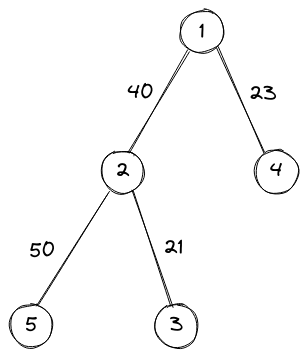# Graph Stuff

Limits 1s, 512 MB

You will be given a tree with $n$ node numbered 1 to $n$ with weighted edge.

You will be asked $q$ queries.

For each query you have to find the largest edge in path from $A$ to $B$.

## Input

The first line contains two integers $n$ and $q$ ($1 \le n, q \le 10^5$). Each of the next $n-1$ lines contains three integer $u_i$, $v_i$, $w_i$, ($1 \le u_i$, $v_i \le n$ and $1 \le w_i \le 10^5$), meaning there is an edge between $u_i$ and $v_i$ with weight $w_i$.

Each of the next $q$ lines contains two integer $A$, $B$ ($1 \le A, B \le n$ and $A \ne B$) describing the query.

## Output

For each query. print the answer in a new line.

## Samples

InputOutput
5 5
2 1 40
3 2 21
4 1 23
5 2 50
1 2
3 5
3 1
2 3
2 1


40
50
40
21
40In query 1, from 1 to 2, there is only one edge and so the answer is 40.

In query 2, from 3 to 5, largest edge in path is 2 -- 5 with weight 50, so the answer is 50.

InputOutput
5 5
2 1 2
3 2 31
4 1 2
5 2 5
1 4
1 5
3 1
2 3
2 1
2
5
31
31
2